# Math Magic in OneNote

## An underutilized OneNote feature is to calculate or solve math equations. Below we show you quick ways to do that:

### 1. Math in its simplest form

Simply type the equation you want to calculate. For example, to calculate the sum of three numbers, type the numbers then the plus sign. To get the sum, just type in the equal sign then hit the space bar (ie:  12+5+23= spacebar). OneNote will then replace the space with the sum (ie:  40).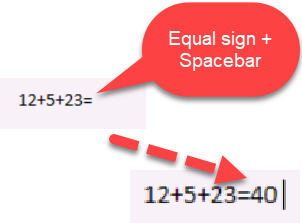### 2. Create Math Equations using Ink or Text with Math Assistant

For this feature you need a valid Microsoft 365 subscription and it will work in;

• OneNote for Microsoft 365
• OneNote for the web
• OneNote for Windows 10 and
• OneNote for iOS.

#### Select the Draw tab

• Select a pen, then select the small arrow that appears next to the pen to choose the stroke width and ink color you want to use.
• If you’re using a touchscreen device, write your equation with your finger or stylus.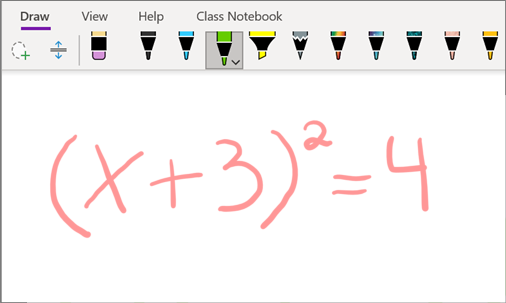• Use your keyboard to type your equation if you have a PC without touchscreen input.

Tip: You can type an equation in linear format and then use the steps below to format it.
Example: (x^2+4)/(x-3)

#### Now Select the Equation

• Select the Lasso Select tool.
• Using your stylus, finger or mouse, drag a line around the equation.
•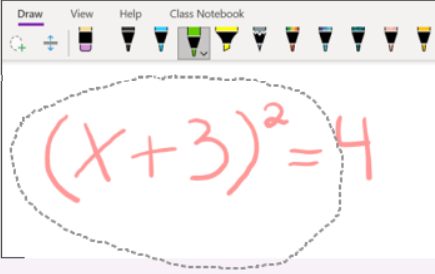Tip: Select Draw with Mouse or Touch to get a better selection result.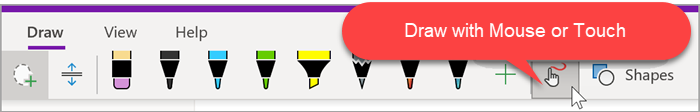#### Finally use the Math button

• Select Math.
• When the Math pane opens, confirm the Equation. If it needs fixing select Fix it.
• Now select Ink to Math to covert your handwriting to a typed math equation.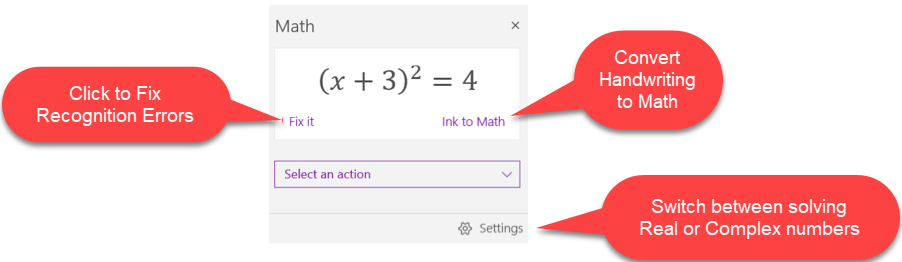### 3. Equations

#### Write an Equation

To write an equation anywhere on a OneNote Page.

• Position your cursor and use “Alt” and “=” keys to bring up the equation editor.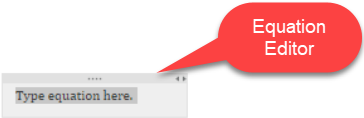• Now you can write simple fractions and equations which OneNote evaluates when you press the Space key.

#### Insert & Edit an Equation

To add a common mathematical equation such as the Area of a Circle or a Fourier Sum. You can also build your equations using the library of math symbols and structure.

• Select the Insert tab, then click Equation
• From the list choose an equation (eg:  Area of Circle)• Use the Equation tab to modify your equationTip: Use the right mouse button context menu to switch between Professional and Liner Equations.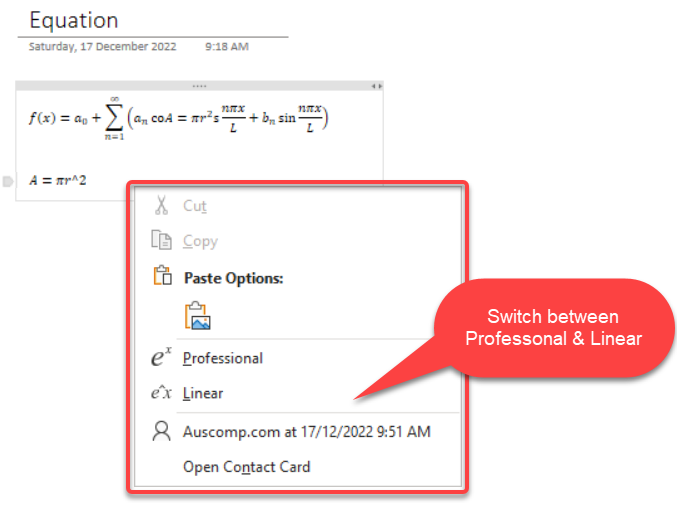### OneNote Planners for Education

Here are planners to put your academic plans on steroids with our Teacher Planners & Ultimate Student Planners,

## Scan documents on the Phone and store them in OneNote

### Welcome to Templates for OneNoteerror: